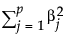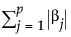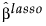An l2 penalty is applied to the regression coefficients during ridge regression. Ridge regression coefficient estimates are given by the following:is the l2 penalty, λ is the tuning parameter, N is the number of rows, and p is the number of variables.
An l1 penalty is applied to the regression coefficients during Lasso. Coefficient estimates for the Lasso are given by the following:is the l1 penalty, λ is the tuning parameter, N is the number of rows, and p is the number of variables
The Elastic Net combines both l1 and l2 penalties. Coefficient estimates for the Elastic Net are given by the following:
 •
 •
 • λ is the tuning parameter
 • α is a parameter that determines the mix of the l1 and l2 penalties
 • N is the number of rows
 • p is the number of variables
For the adaptive Lasso, this weighted form of the l1 penalty is used in determining thecoefficients.
The adaptive Elastic Net uses this weighted form of the l1 penalty and also imposes a weighted form of the l2 penalty. The weighted form of the l2 penalty for the adaptive Elastic Net is

Help created on 9/19/2017